# How to calculate percentage of total in Excel?

In this article, we are going to go over a few methods that will assist you in calculating percentages in Excel in an effective manner, as well as understand the fundamental percentage formulas that will remove the element of guessing from your calculations.

To determine the percentage, you can either press "Ctrl+Shift+%" or select the "%" button in the number group of the Home tab in order to convert the output to a percentage.

The illustration that was just given is a specific instance of calculating percentages of a total. Now, let's study a few more examples that will help you make quick work of computing a percentage of a total in Excel on various data sets. This example will be helpful since they will demonstrate how to do so in a variety of scenarios.

Let’s understand step by step with an example.

## Step 1

In the first step, we must create sample data. As shown in the below screenshot.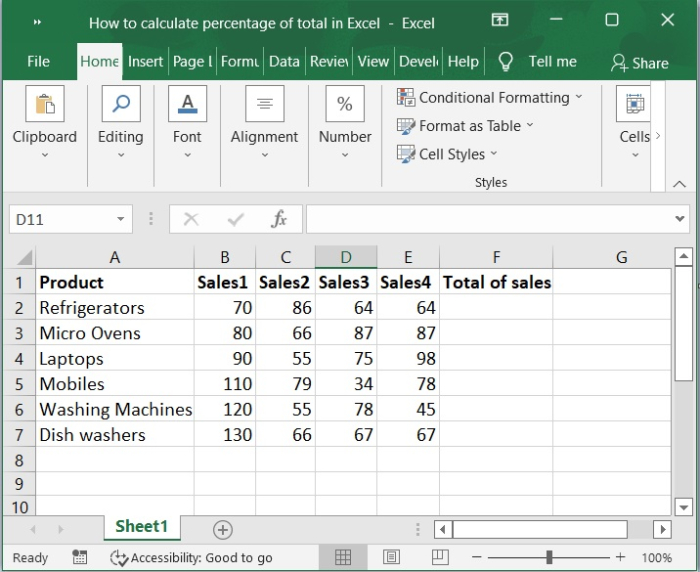## Step 2

Now, you need to enter the formula in the cell(F2) total of sales.

=SUM(B2:E2)


Here, B2 and E2 represent sales.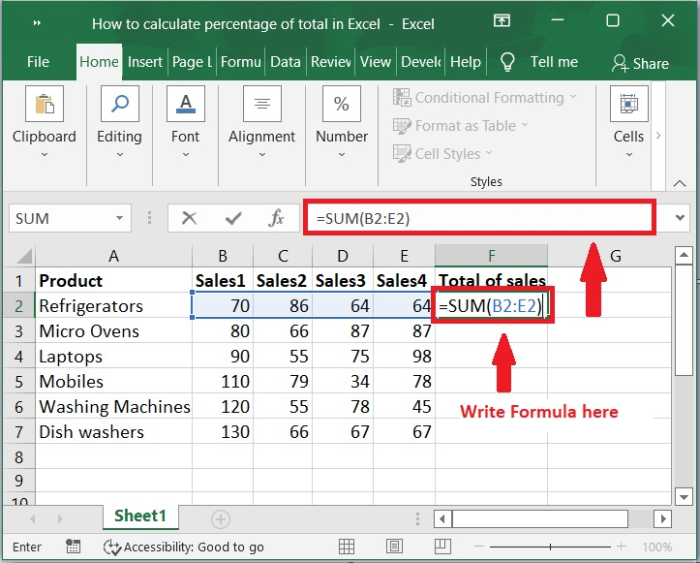## Step 3

After that, the total of sales will appear in the cell F2. Now drag to fill handle to the bottom of its range. Check out the below screenshot for the same.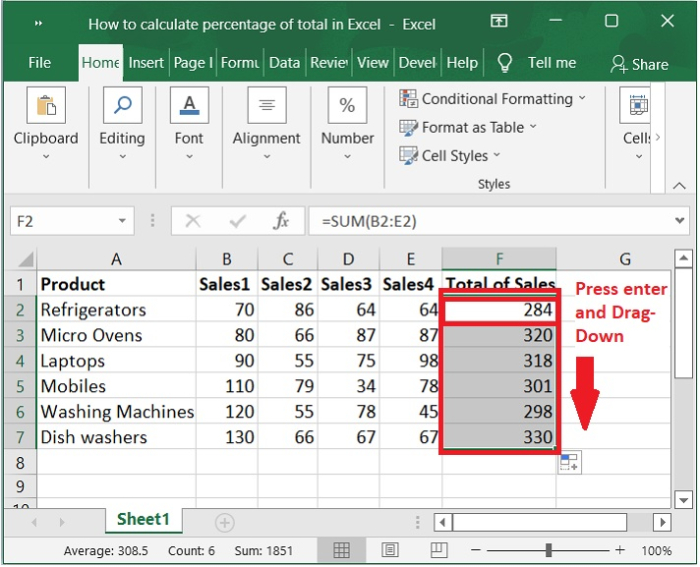## Step 4

Choose Cell F8 and enter the formula to calculate the total of sales total. Then press Enter key. As shown in the below screenshot.

=SUM(F2:F7)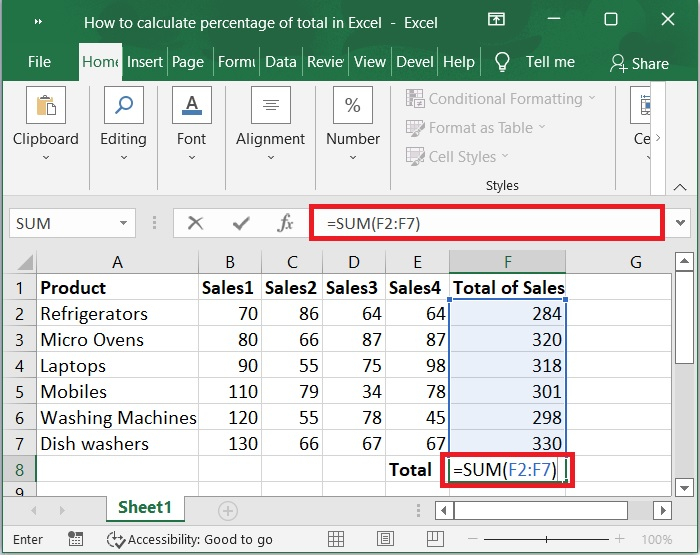## Step 5

Now, you can calculate the percentage of total by using the formula given below.

=F2/$F$8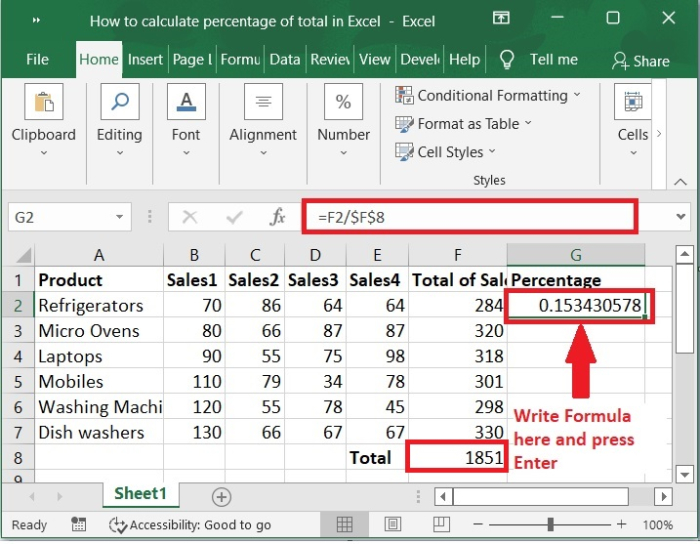## Step 6

Now drag the fill handle down till you want to calculate the percentage. As shown in the below screenshot.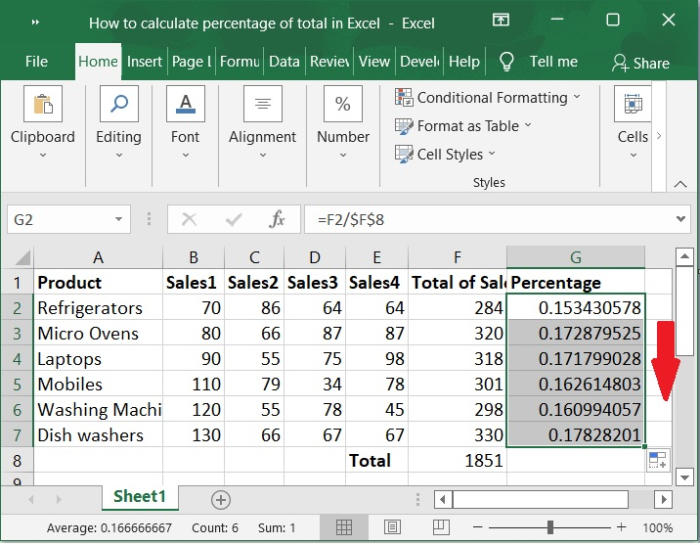## Step 7

To get the values in percentage format, select the data and click Home tab and select Number group choose % symbol so that cells will get formatted. Shortcut press "Ctrl+Shift+%".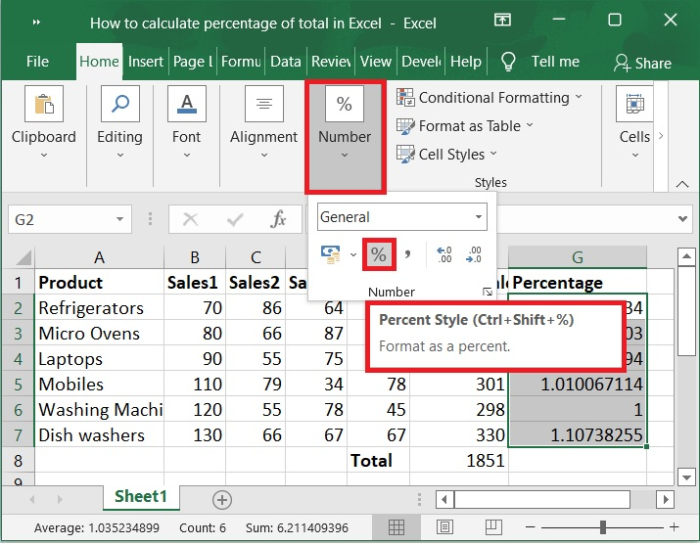## Step 8

Once you click on the percentage (%) symbol. You will get the values in % format as shown in the below screenshot.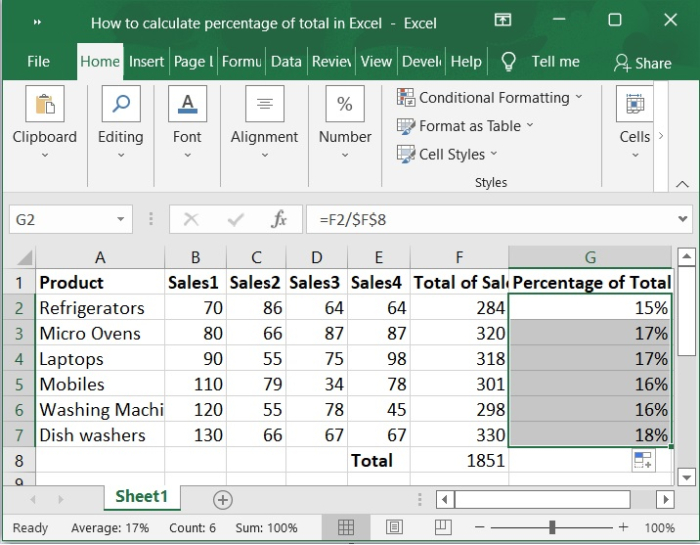## Conclusion

We hope this tutorial helped you understand how to calculate the percentage of total by using formulas and percentage symbols in Excel.

Updated on: 03-Feb-2023

182 Views